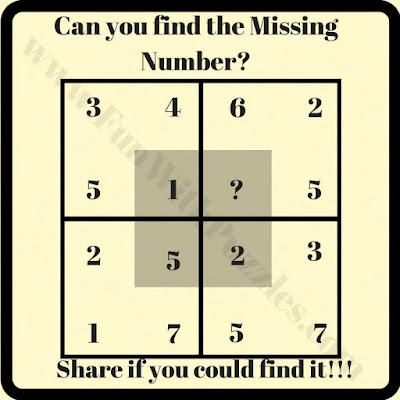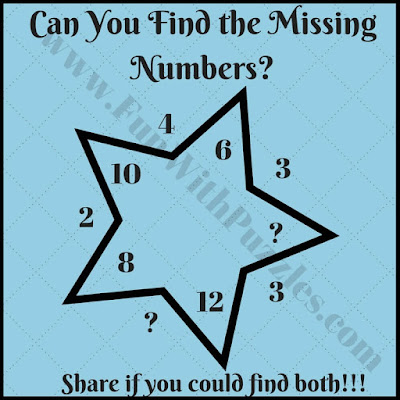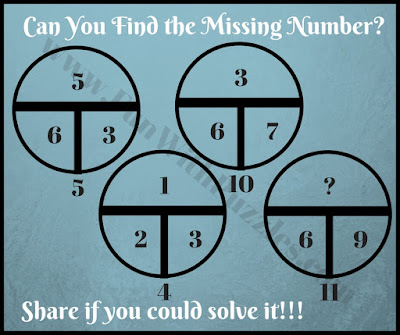For the last few weeks, we have been publishing the different types of Math Brain Teasers. Most of these brain teasers were based on one Geometrical shape. In these puzzles, this shape contains a few numbers around it which are mathematically related to each other. One has to find this mathematical relationship or pattern to solve it for the missing digit. In this post, we are going to give one maths logic puzzle of each type and then link to the other similar puzzles given at the end of each puzzle.

## How to Solve Mind-Bending Maths Puzzles and Brain Teasers

In these puzzles, some numbers around one shape are given. These numbers are related to each other mathematically. Your challenge in these mind-bending math puzzles is to crack the hidden mathematical pattern among these numbers. Once you are able to crack the code, then find the missing number which will replace the question mark in each puzzle.

These are tough puzzles. However, a webpage link after each puzzle will lead to similar puzzles which are easy to solve. So don't forget to check out linked puzzles if you find any of these puzzle types interesting as there are many puzzles waiting to be discovered by you.1. Mind-Bending Math Cross-Circle Number Puzzle Question2. Tough Picture Math Circle Brain Teaser3. Tough Picture Math Square Puzzle Question4. Tricky Picture Math Star Number Puzzle Question5. Very Tough Picture Math Circle Brain Teaser

The answer to some of these "Mind-Bending Maths Puzzles and Brain Teasers for Adults", can be viewed by clicking on the button. Please give your best try before looking at the answers.

Anonymous said...

Pl supply answers if there are any and u know them

Fun With Puzzles said...

Answers of most of these Mind Bending Tough Puzzles is given. You need to click on the "View Answer" button to read the answers for these puzzles. Do let us know in case something is not clear in the explanation of these answers for any of these Mind Bending Puzzles?

Unknown said...

1

Dee said...

#2. 2